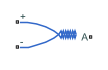# Thermocouple

Sensor that converts thermal potential difference into electrical potential difference

•Libraries:
Simscape / Electrical / Sensors & Transducers

## Description

The Thermocouple block represents a thermocouple using the standard polynomial parameterization defined in the NIST ITS-90 Thermocouple Database .

For thermocouples Type B, E, J, K (t<=0 degC), N, R, S or T, the voltage E across the device in mV is

`$E\left(mV\right)={c}_{0}+{c}_{1}t+...+{c}_{n}{t}^{n}$`

where:

• ci is the ith element of the Coefficients [c0 c1 ... cn] parameter value.

• t is the temperature difference in degrees Celsius between the temperature at the thermal port A and the Reference temperature parameter value.

Note

The equation for voltage across the device as a function of temperature difference is defined in mV. The units of the voltage across the actual device is V.

For thermocouples Type K (t>=0 degC), the equation contains an additional exponential term:

`$E\left(mV\right)={c}_{0}+{c}_{1}t+...+{c}_{n}{t}^{n}+{a}_{0}{e}^{{a}_{1}{\left(t-{a}_{2}\right)}^{2}}$`

where a0, a1, and a2 are additional coefficients, required only by the Type K thermocouple, defined by the Coefficients [a0 a1 a2] parameter value.

The following equation describes the thermal behavior of the block:

`$Q={K}_{d}{t}_{c}\frac{dT}{dt}$`

where:

• T is the temperature at port A.

• Q is the net heat flow into port A.

• Kd is the Dissipation factor parameter value.

• tc is the Thermal time constant parameter value.

• dT/dt is the rate of change of the temperature.

To model the thermocouple in free space:

1. Connect the thermocouple to the B port of a Simscape™ Convective Heat Transfer block.

2. Connect the A port of the Convective Heat Transfer block to a Simscape Ideal Temperature Source block whose temperature is set to the ambient temperature.

3. Set the Area parameter of the Convective Heat Transfer block to an approximate area Anom.

4. Set the Heat transfer coefficient parameter of the Convective Heat Transfer block to Kd/Anom.

## Assumptions and Limitations

• The high-order polynomials this block uses are very sensitive to the number of significant figures used for computation. Use all available significant figures when specifying the Coefficients [c0 c1 ... cn] parameter.

• Coefficients [c0 c1 ... cn] are defined for use over a specified temperature range.

• The maximum supported value for `n` in the Coefficients [c0 c1 ... cn] parameter is 14, that is, the vector cannot have more than 15 elements.

## Ports

### Conserving

expand all

Thermocouple thermal port.

Electrical conserving port associated with the thermocouple positive port.

Electrical conserving port associated with the thermocouple negative port.

## Parameters

expand all

### Electrical

Select one of the modeling options:

• ```Type B, E, J, K (t<=0 degC), N, R, S or T``` — This option is equivalent to the block functionality in previous releases.

• `Type K (t>=0 degC)` — This option adds an exponential term to the block equations when the temperature difference is greater than 0 degrees Celsius.

Vector of coefficients c in the equation that describes voltage as a function of temperature. The maximum length of the vector is 15 elements.

Note

You can download parameters for standard thermocouple types from the NIST database . For information on how to do this, see the Simulink®Approximating Nonlinear Relationships: Type S Thermocouple example.

Vector of additional coefficients a0, a1, and a2, required only by the Type K thermocouple.

#### Dependencies

This parameter is visible only when you select ```Type K (t>=0 degC)``` for the Thermocouple model parameter.

### Thermal

Temperature the block subtracts from the temperature at the thermal port in calculating the voltage across the device.

Time it takes the thermocouple temperature to reach 63% of the final temperature change when a step change in ambient temperature occurs.

Thermal power required to raise the thermocouple temperature by one K.

Temperature of the thermocouple at the start of the simulation.

 Dean C. Ripple, NIST ITS-90 Thermocouple Database, December 21, 1999 (https://srdata.nist.gov/its90/main/)